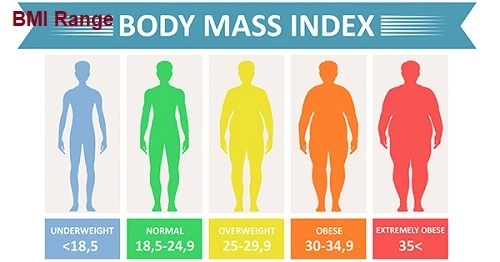# BMI Range (From underweight value – Obese value)## BMI RANGE

In this article we will be talking  about BMI range. Before we get there, let us have a brief overview on what BMI is.

BMI which is an acronym for Body Mass Index is a standard use to figure out a healthy weight for your height. In general, the higher the BMI, the more body fat a person has and vice versa. It is often used as a screening tool to decide if your weight might be putting you at risk for health problems such as heart disease, diabetes, and cancer.

## BMI range (Standard values)

BMI range states the range of values which determines if a person is under weight, normal weight, over weight or obese.

Below is the BMI range. The range is categorized into, under weight, normal weight, over weight and obese.

• Underweight: BMI is less than 18.5
• Normal weight: BMI is 18.5 to 24.9
• Overweight: BMI is 25 to 29.9
• Obese: BMI is 30 or more

Or

• Underweight: 124 lbs or less
• Normal weight: 125 lbs to 168 lbs
• Over weight: 169 lbs to 202 lbs
• Obese: 203 lbs or more

For most adults, an ideal BMI is in the 18.5 to 24.9 range.

## BMI range in children and teens

The US Centers for Disease Control and Prevention (CDC) has developed age and gender specific growth charts. These charts are used to translate a BMI number into a percentile based on a child’s sex and age.

This is because in children and teens, their BMI depends on factors like age, and sex; as the normal amount of body fat changes with age in children and teens, and is also different between boys and girls.

The percentiles are then used to determine the different weight groups:

• Underweight: less than the 5th percentile
• Normal weight: 5th percentile to less than the 85th percentile
• Overweight: 85th percentile to less than the 95th percentile
• Obese: 95th percentile or higher

An easy way to determine your child’s BMI percentile is to use the CDC’s online BMI percentile calculator at https://www.cdc.gov/healthyweight/bmi/calculator.html.

## How to calculate BMI

To determine the BMI range of an individual, i.e, if he/she is within the normal range, you must first determine the BMI by calculation.

Calculation formula

Formula: weight (kg) / [height (m)]2

With the metric system, the formula for BMI is weight in kilograms divided by height in meters squared. Because height is commonly measured in centimeters, divide height in centimeters by 100 to obtain height in meters.

Example: Weight = 68 kg, Height = 165 cm (1.65 m)
Calculation: 68 ÷ (1.65)2 = 24.98

Or

Formula: weight (lb) / [height (in)]2 x 703

Calculate BMI by dividing weight in pounds (lbs) by height in inches (in) squared and multiplying by a conversion factor of 703.

Example: Weight = 150 lbs, Height = 5’5″ (65″)
Calculation: [150 ÷ (65)2] x 703 = 24.96

## Consequence of obesity in adult

Some of the consequences of obesity include:

• High blood pressure (Hypertension)
• High LDL cholesterol, low HDL cholesterol, or high levels of triglycerides (Dyslipidemia)
• Type 2 diabetes
• Coronary heart disease
• Stroke
• Osteoarthritis (a breakdown of cartilage and bone within a joint)
• Sleep apnea and breathing problems
• Chronic inflammation and increased oxidative stress19,20
• Some cancers (endometrial, breast, colon, kidney, gallbladder, and liver), etc.

This is why we should also check our BMI and ensure that it is within the normal range. This is the only way we can avoid the health issues associated with high BMI.